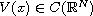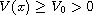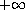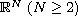Electron. J. Diff. Equ., Vol. 2014 (2014), No. 252, pp. 1-12.

### Nontrivial solutions for asymmetric problems on R^N Ruichang Pei, Jihui Zhang

Abstract:
We consider the elliptic equationwhereandfor all. The nonlinear termexhibits an asymmetric growth atandin. Namely, it is linear atand superlinear at. However, it need not satisfy the Ambrosetti-Rabinowitz condition on the positive semiaxis. Some existence results for nontrivial solution are established by using the minimax methods combined with the improved Moser-Trudinger inequality.

Submitted October 5, 2014. Published December 4, 2014.
Math Subject Classifications: 35J60, 35J20, 35B38.
Key Words: Schrodinger equation; asymmetric problems; one side resonance; subcritical exponential growth.

Show me the PDF file (253 KB), TEX file, and other files for this article.Ruichang Pei School of Mathematics and Statistics Tianshui Normal University Tianshui 741001, China email: prc211@163.com Jihui Zhang School of Mathematics and Computer Sciences Nanjing, Normal University Nanjing 210097, China email: zhangjihui@njnu.edu.cn